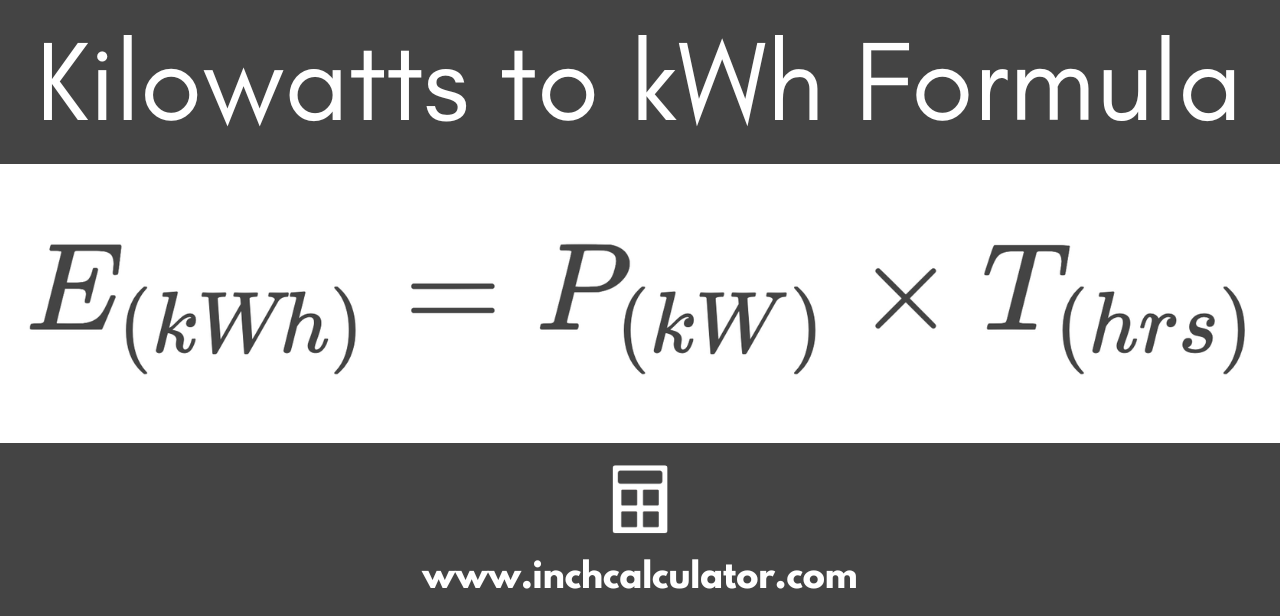# Kilowatts (kW) to Kilowatt-Hours (kWh) Conversion Calculator

Convert kilowatts to kilowatt-hours by entering the power in kW and the time in hours below.

## Energy in Kilowatt-Hours:

kWh
Learn how we calculated this below

## How to Convert Kilowatts to Kilowatt-Hours

Kilowatts, or kW, are a measure of electrical power. Kilowatt-hours, abbreviated as kWh or kW·h, are a measure of energy used. One kilowatt-hour is equal to one kilowatt of power consumed over a one-hour time period.

### kW to kWh Conversion Formula

To convert power in kW to energy in kWh, use the formula below.

E(kWh) = P(kW) × T(hrs)

The energy E in kilowatt-hours is equal to the power P consumed in kilowatts times the time T in hours.For example, let’s convert 25 kW power used over a 4-hour time period to energy in kWh.

EkWh = 25 kW × 4 hours
EkWh = 100 kWh

So, 25 kW of power consumed over 4 hours is equal to 100 kWh of energy.

Do you want to convert kWh to kW?

## Common Kilowatt to Kilowatt-Hour Conversions

Table showing the energy in kilowatt-hours to the power in kilowatts for various lengths of time.
Power (in kW) Time (in hours) Energy (in kWh)
1 kW 1 hrs 1 kWh
1 kW 2 hrs 2 kWh
1 kW 3 hrs 3 kWh
1 kW 4 hrs 4 kWh
1 kW 5 hrs 5 kWh
1 kW 6 hrs 6 kWh
1 kW 7 hrs 7 kWh
1 kW 8 hrs 8 kWh
1 kW 9 hrs 9 kWh
1 kW 10 hrs 10 kWh
1 kW 15 hrs 15 kWh
1 kW 20 hrs 20 kWh
1 kW 25 hrs 25 kWh
1 kW 30 hrs 30 kWh
1 kW 35 hrs 35 kWh
1 kW 40 hrs 40 kWh
1 kW 45 hrs 45 kWh
1 kW 50 hrs 50 kWh
1 kW 55 hrs 55 kWh
1 kW 60 hrs 60 kWh
1 kW 65 hrs 65 kWh
1 kW 70 hrs 70 kWh
1 kW 75 hrs 75 kWh
1 kW 80 hrs 80 kWh
1 kW 85 hrs 85 kWh
1 kW 90 hrs 90 kWh
1 kW 95 hrs 95 kWh
1 kW 100 hrs 100 kWh

Try our watts to kWh calculator.